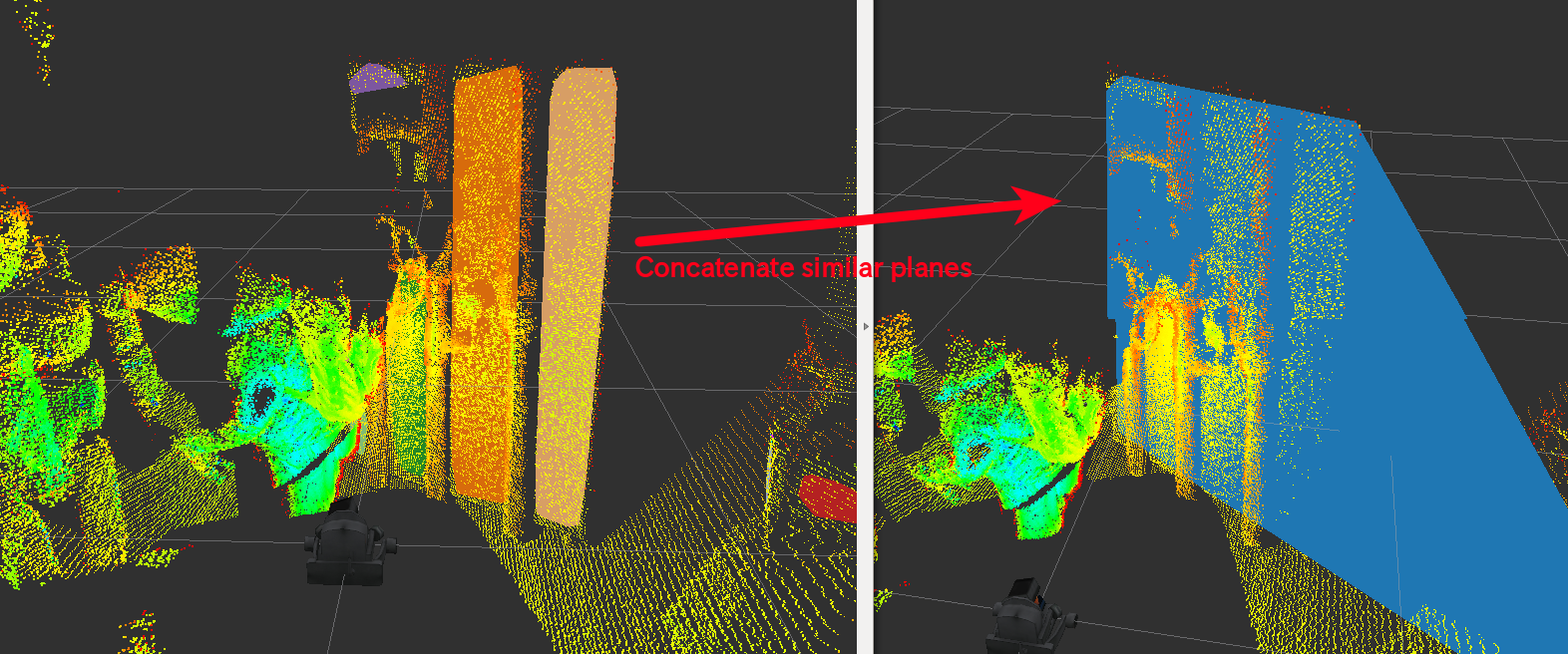# PlaneConcatenator¶Concatenate near planes and build new set of planes.

## Subscribing Topic¶

• ~input (sensor_msgs/PointCloud2)

Input pointcloud.

• ~input/indices (jsk_recognition_msgs/ClusterPointIndices)

• ~input/polygons (jsk_recognition_msgs/PolygonArray)

• ~input/coefficients (jsk_recognition_msgs/ModelCoefficientsArray)

Input planes.

## Publishing Topics¶

• ~output/indices (jsk_recognition_msgs/ClusterPointIndices)

• ~output/polygons (jsk_recognition_msgs/PolygonArray)

• ~output/coefficients (jsk_recognition_msgs/ModelCoefficientsArray)

Concatenated planes. Coefficients parameters are refined by RANSAC. If the coefficients are not concatenated, they will be output as is, but if they are refined by RANSAC, they will be forced to face the origin of the frame.

## Parameters¶

• ~connect_angular_threshold (Double, default: 0.1)

Angular threshold to regard two planes as near.

• ~connect_distance_threshold (Double, default: 0.1)

Euclidean distance threshold to regard two planes as near.

• ~connect_perpendicular_distance_threshold (Double, default: 0.1)

Distance threshold to connect two planes in perpendicular direction.

• ~ransac_refinement_max_iteration (Integer, default: 100)

The maximum number of iteration of RANSAC refinement.

• ~ransac_refinement_outlier_threshold (Double, default: 0.1)

Outlier threshold of RANSAC refinmenet.

• ~ransac_refinement_eps_angle (Double, default: 0.1)

Eps angle threshold of RANSAC refinment using normal direction of the plane.

• ~min_size (default: 100)

Minimum inlier of concatenated polygons.

• ~min_area (default: 0.1)

• ~max_area (default: 100.0)

Minimum and maximum area of concatenated polygons.

## Sample¶

roslaunch jsk_pcl_ros_utils sample_plane_concatenator.launch